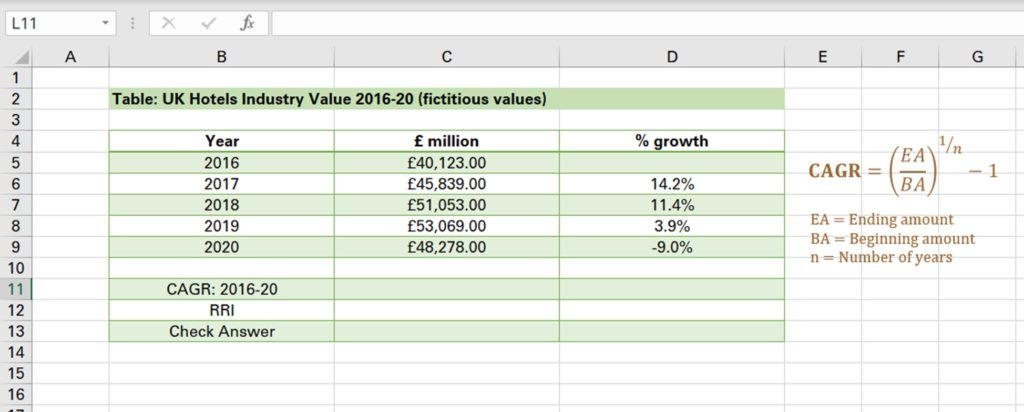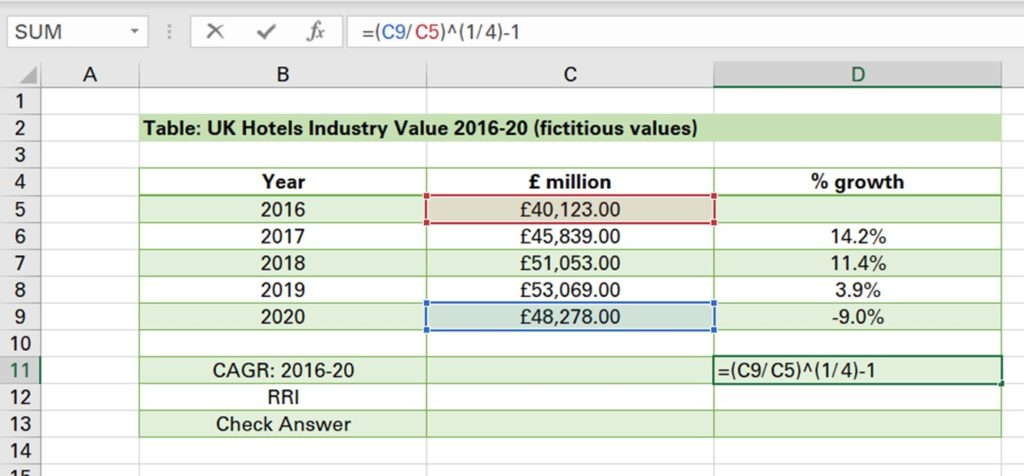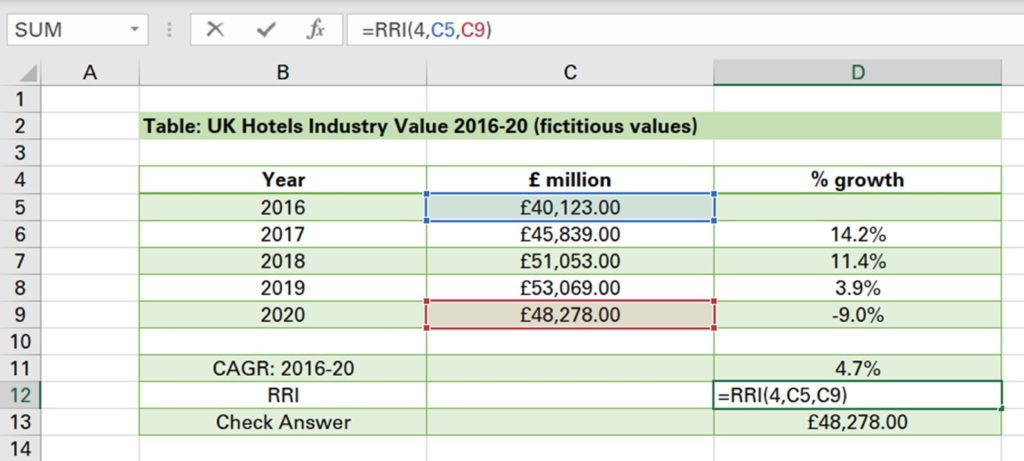## How to calculate cagr

The Compound Annual Growth Rate (CAGR) calculates the rate of return over a period of time and it is often used to measure past performance of investments or analyse business measures like sales or market value. In our example, we will calculate the compounded annual rate of growth of the UK hotels industry.

We have the fictitious industry values for the years 2016 to 2020. We also have the individual growth rates year-on-year. However, the problem with these figures is that they’re uneven which makes it difficult to deduce what the general trend in industry value was between 2016-2020. To overcome this, we can calculate the CAGR which shows what the growth rate would be if it were constant every single year.There are two methods to calculate this. Firstly, we can manually calculate the CAGR using the formula above. We take the ending amount which is the 2020 industry value and divide the beginning amount which is the 2016 industry value. This is to the power of the inverse of the number of years, so ¼, close brackets and then subtract 1. Be careful with the number of years, even though there are 5 years listed, it is 4 years from the end of 2016 to 2020. Whenever in doubt simply take the end year which is 2020 minus the start year which is 2016 and this gives the number of compounding years which equals 4.

Therefore, the CAGR is 4.7%. In other words, if the industry value had increased by a constant rate of 4.7% every year, then the value in 2020 would be 48,278 pounds.So that’s how you can manually calculate the CAGR in excel. However, there is a function which makes things a bit easier. This is the RRI function which was specifically introduced to calculate CAGR. So we type = RRI, first enter the number of periods which is 4, then input the present value or beginning amount which is £40,123 followed by the ending amount which is £40,278 and click enter. This gives the exact same answer.Now, how do we check whether the answer is correct? Well we can take the beginning amount and multiply it by 1 plus the CAGR to the power of 4. This gives the 2020 value. In other words, if in 2017 the industry value grows by 4.7%, in 2018 it grows by 4.7%, in 2019 it grows by THIS AMOUNT and in 2020 it grows by 4.7% then the value in 2020 would be £48,278 which equals the 2020 value.